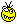# Earth destruction: Meteorite threat assessment

Lately some of internet community was shocked by a missed 7 meter meteorite, which was detected just 15 hours before minimal distance to Earth, and that showed that we are still under risk of going Dinosaur way – have a breakfast, and then suddenly die due to meteorite impactSome might say "Hey, this 7 meter meteorite is so tiny to cause any damage". I am not going just believe that, let's find out by ourselves!

I'll show you that meteorite damage assessment is not something available for eggheads only: you might find all required information and tools for that in the internet, and I'll show you how :-)

The only thing you should remember from School physics course is that every moving body have kinetic energy, and whatever you do with that body – amount of energy is preserved. I.e. when meteorite hit the Earth – this energy gets converted to heat of explosion and shock-wave.

## Meteorite's mass

Google "Meteorite density", in the first link you'll find out that usual meteorite density is 3 grams per cubic centimeter, or 3 tons per cubic meter (Sorry, no imperial units here). Diameter of recent missed meteorite was 7 meters.
I guess you do not remember formula for sphere volume? Not a big deal.Go to http://www.wolframalpha.com/index.html , enter "Sphere volume", key into our value (radius 3.5m) – and we are getting volume of 180 cubic meters, and mass 180*3 = 540 tons. Looks bigger now?## Calculating kinetic energy

We'll need to find out average meteorite speed: http://en.wikipedia.org/wiki/Meteorite">http://en.wikipedia.org/wiki/Meteorite We see that usual meteorite speed is 11-25 km/second, let's take 20 km/sec for our calculations.
Go to http://www.wolframalpha.com/index.html enter "kinetic energy", enter data we have (mass and speed) and we've got meteorite kinetic energy. It's 1.08*1014 Joules.

This is the amount of energy will be released when meteorite hits the Earth, no matter will it burn down in atmosphere, melt or vaporize. Also, Earth's atmosphere is quite thin (at least dense part), and at the speed 20km/s meteorite would pass it and hit the Earth in 1-2 seconds (It's not like in movies). If meteorite is coming under narrow angle to the Earth's surface – then this energy would be released over larger area, and the impact would be lower, but the amount of released energy is still exactly the same.

Even if meteorite (unlikely) explodes (vaporizes almost instantly) at the great height, it still might cause destruction on the Earth surface due to shockwave (for big meteorites it might go 10km and further).
A word about melting/slowing down/vaporizing of meteorites: air resistance rises as square of size, but kinetic energy/meteorite mass as a cube. That means bigger meteorite - easier atmosphere pass.

## Converting to Megatons

Let's compare that energy to an nuclear explosion. Open, http://en.wikipedia.org/wiki/Nuclear_weapon_yield
Here we see that 1 megaton (Mt) is 4,184x1015 Joules, so that 7 meter meteorite would release energy equivalent to 25 kiloton nuclear explosion (or explosion of 25'000 tonns of TNT).

## Material damage

Go to http://en.wikipedia.org/wiki/Effects_of_nuclear_explosions :
Here we see that for 20kt explosion zone of complete destruction have radius of 1km, 20Mt – 10km, 100Mt – 35km. You should remember that you may see severe damage of civilian buildings up to 5x of that range.

## Judgment day meteorite

Have you ever interested how big should be a meteorite to kill most of life forms on the Earth? (especially human beings)
Let's assume that to achieve that we need to heat all air over the Earth by 100 degrees (celsium), and heat top 20 meters of water by 50 degrees. Surely it will cool down quickly (hours-days) but that wouldn't matter then for everyone except ISS astronautsHeating air:
At the http://en.wikipedia.org/wiki/Earth's_atmosphere we may find weight of all air on the Earth, it's 5.2*1018 kg, it's heat capacity is ~1000 J/(kg/degree). So, to heat up all Earth's atmosphere by 100C, we need 5.2*1023 Joules of energy.
Heating water:
At the http://en.wikipedia.org/wiki/Earth, we see that Earth's surface area is 510,072,000 km2, to get square meters we need to multiply that by 1'000'000. Heat capacity of water is ~4180 J/(kg/degree), so to heat up 20 meters layer of water over all Earth's surface by 50 degrees we would need 510'072'000'000'000(Earth's surface)*1000(how many kilograms per cubic meter of water)*20(thikness)*50(degrees)*4018(heat capacity) = 2.049*1023 Joules of energy.
Total:
Totally we need 7.25*1023 Joules. Now go back to http://www.wolframalpha.com/index.html , enter "kinetic energy" and find out meteorite mass for that energy. It appears to be 3.625*1015 kg.
Divide that by meteorite density, and we are getting meteorite volume of 1.2*1012 m2.
And finally, enter "Sphere volume" and calculate radius/diameter of meteorite of that volume:
Judgment meteorite which is able to destroy all life over the Earth appears to have diameter of 13.2 kilometers.

## Summary

After repeating these computations for a few times, we might get that table:
 Meteorite diameter Blast yield (Mt) Radius of complete destruction zone 7m 0.025 1km 64m 20 10km 110m 100 35km 1000m 75'09013200m 173'000'000Judgement day meteorite